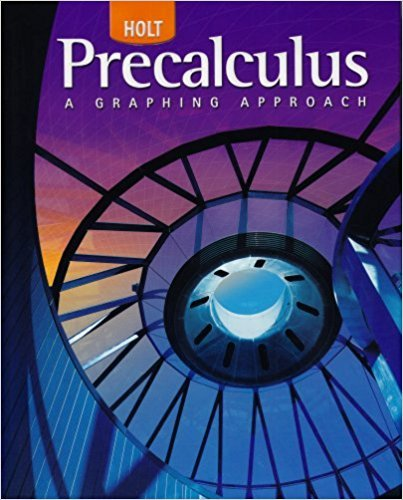×
×

# Solutions for Chapter 11.5: Analytic Geometry## Full solutions for Precalculus | 1st Edition

ISBN: 9780030416477Solutions for Chapter 11.5: Analytic Geometry

Solutions for Chapter 11.5
4 5 0 290 Reviews
24
5
##### ISBN: 9780030416477

Precalculus was written by and is associated to the ISBN: 9780030416477. This expansive textbook survival guide covers the following chapters and their solutions. Since 16 problems in chapter 11.5: Analytic Geometry have been answered, more than 23534 students have viewed full step-by-step solutions from this chapter. This textbook survival guide was created for the textbook: Precalculus, edition: 1. Chapter 11.5: Analytic Geometry includes 16 full step-by-step solutions.

Key Calculus Terms and definitions covered in this textbook
• Angular speed

Speed of rotation, typically measured in radians or revolutions per unit time

• Arctangent function

See Inverse tangent function.

• Axis of symmetry

See Line of symmetry.

• Compounded monthly

See Compounded k times per year.

• Confounding variable

A third variable that affects either of two variables being studied, making inferences about causation unreliable

• Dot product

The number found when the corresponding components of two vectors are multiplied and then summed

• Equilibrium price

See Equilibrium point.

• Identity function

The function ƒ(x) = x.

• Linear regression equation

Equation of a linear regression line

• n-set

A set of n objects.

• Negative angle

Angle generated by clockwise rotation.

• Number line graph of a linear inequality

The graph of the solutions of a linear inequality (in x) on a number line

• Perihelion

The closest point to the Sun in a planet’s orbit.

• Placebo

In an experimental study, an inactive treatment that is equivalent to the active treatment in every respect except for the factor about which an inference is to be made. Subjects in a blind experiment do not know if they have been given the active treatment or the placebo.

• Quotient of complex numbers

a + bi c + di = ac + bd c2 + d2 + bc - ad c2 + d2 i

• Reciprocal identity

An identity that equates a trigonometric function with the reciprocal of another trigonometricfunction.

• Semimajor axis

The distance from the center to a vertex of an ellipse.

• Sphere

A set of points in Cartesian space equally distant from a fixed point called the center.

• Variable (in statistics)

A characteristic of individuals that is being identified or measured.

• xz-plane

The points x, 0, z in Cartesian space.

×